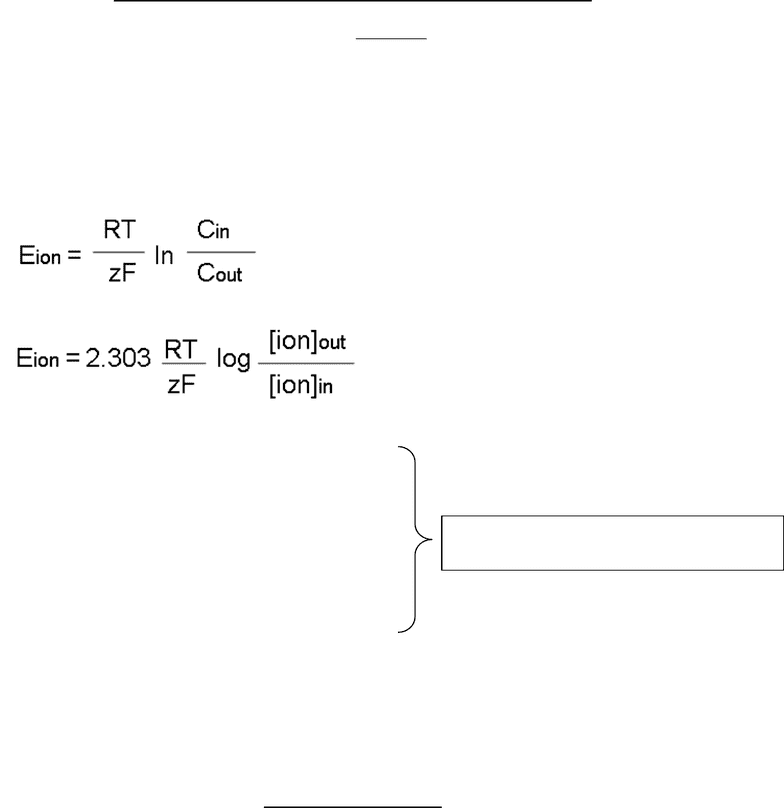Class Notes (1,100,000)
CA (620,000)
Brock U (10,000)
BIOL (1,000)
drfu (30)
Lecture 17

# BIOL 2P98 Lecture Notes - Lecture 17: Nernst Equation, Reversal Potential, Gas Constant

Department
Biological Sciences
Course Code
BIOL 2P98
Professor
drfu
Lecture
17

This preview shows page 1. to view the full 4 pages of the document.HLSC 2P09 1
Seminar Four: Equilibrium, Nernst, and Membrane Potential
Part 1: Nernst or Equilibrium Potential (for a single ion across the cell membrane):
See p.160-166 in Silverthorn textbook
The Nernst potential or Equilibrium potential of an ion is the potential across the membrane that
prevents net diffusion of the ion in either direction through the membrane.
The Nernst Equation is also known as the Equilibrium Potential or Eion and is as follows:
Eion = the equilibrium potential of an ion
R = is the universal gas constant (8.314 Jmol-1K-1)
T = temperature in Kelvin (K) at 310K (body temp)
Z = valence of the ion
F = Faradays constant (9.64853 x 104 coulombs/mole)
[ion]out is the ion concentration outside the cell
[ion]in is the ion concentration inside the cell
2.303 = conversion factor between ln & log
(𝟐. 𝟑𝟎𝟑)(𝟖. 𝟑𝟏𝟒)(𝟑𝟏𝟎)
(𝟗. 𝟔𝟒𝟖𝟓𝟑 𝒙 𝟏𝟎𝟒)
= 𝟎. 𝟎𝟔𝟏𝑽 𝒐𝒓 𝟔𝟏𝒎𝑽
V= volts mV=millivolts (1mV = 10-3 V)
The sum of 2.303RT/zF gives you a value of 61
###### You're Reading a Preview

Unlock to view full version